Select Page

# CBSE Maths 12 Science MCQ Integrals Solutions in English

CBSE Maths 12 Science MCQ Integrals Solutions in English to enable students to get Solutions in a narrative video format for the specific question.

Expert Teacher provides CBSE Maths 12 Science MCQ Integrals Solutions through Video Solutions in English language. This video solution will be useful for students to understand how to write an answer in exam in order to score more marks. This teacher uses a narrative style for a question from Integrals not only to explain the proper method of answering question, but deriving right answer too.

Please find the question below and view the Solution in a narrative video format.

Question:

Solution Video in English:

## Similar Questions from CBSE, 12th Science, Maths, Integrals

Question 1 : Evaluate :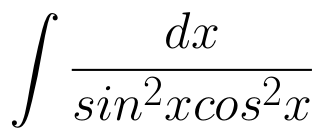. (View Answer Video)

Question 2 : Find: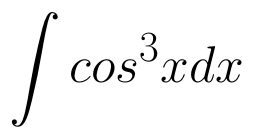. (View Answer Video)

Question 3 :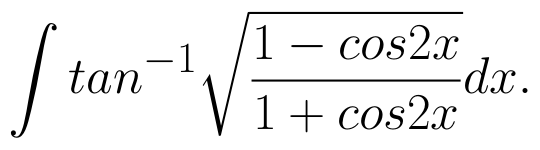(View Answer Video)

Question 4 : Find: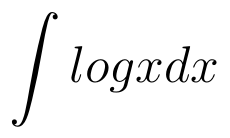. (View Answer Video)

Question 5 : Prove that :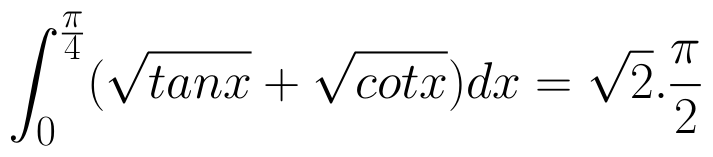(View Answer Video)

### Application of Integrals

Question 1 : Find the area between the curves y = x and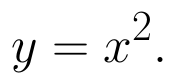(View Answer Video)

Question 2 : Find the area bounded by the curve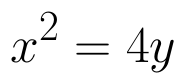and the line x = 4y - 2. (View Answer Video)

Question 3 : Using integration, find the area of the region bounded by the curves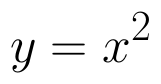and y = x. (View Answer Video)

Question 4 : Using the method of integration find the area of the region bounded by lines:
2x + y = 4, 3x - 2y = 6 and x - 3y + 5 = 0.

Question 5 : Using integration, find the area of the triangle formed by a positive x-axis and tangent and normal to the circle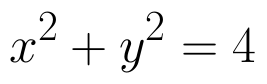at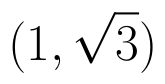. (View Answer Video)

### Application of Derivatives

Question 1 : The total revenue in rupees received from the sale of x units of a product is given by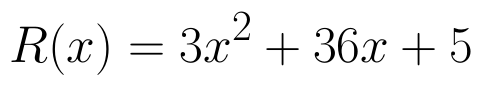. The marginal revenue, when x=15 is: (View Answer Video)

Question 2 : It is given that  at x=1, the function attains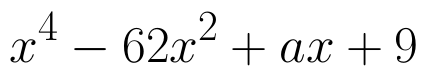its maximum value on the interval[0,2]. Find the value of a? (View Answer Video)

Question 3 : Find two positive numbers whose sum is 16 and the sum of whose cubes is minimum. (View Answer Video)

Question 4 : Find two positive numbers x  and y such that x+y=60 and is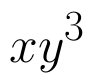maximum. (View Answer Video)

Question 5 : Find the approximate change in volume V of a cube of side x meters caused by increasing the side by 1%. (View Answer Video)

### Differential Equations

Question 1 : Write the degree of the differential equation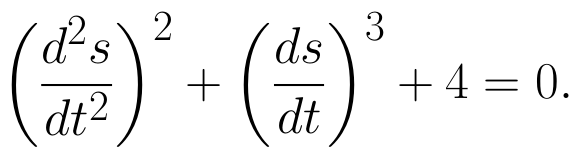(View Answer Video)

Question 2 : If x cos(a + y) = cos y, then prove that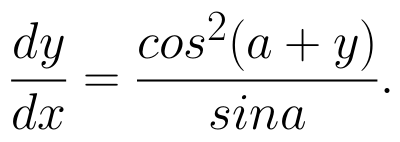Hence show that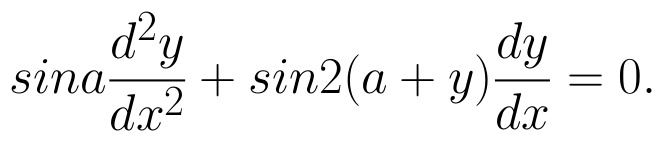(View Answer Video)

Question 3 : Form the differential equation of equation y = a cos 2x + b sin 2x, where a and b are constant. (View Answer Video)

Question 4 : Determine degree of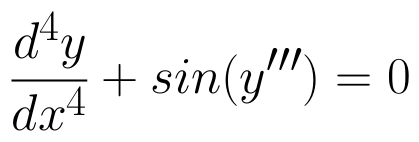(View Answer Video)

Question 5 : If y(x) is a solution of the differential equation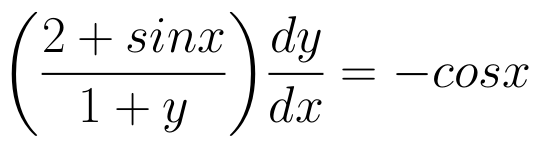and y(0) = 1, then find the value of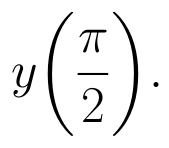(View Answer Video)